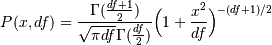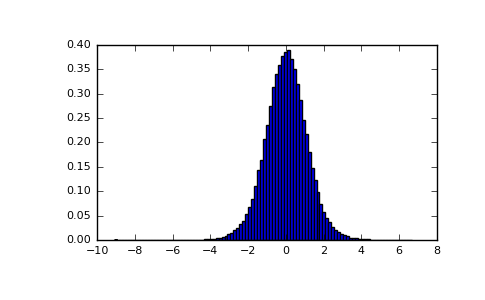# numpy.random.standard_t¶

numpy.random.standard_t(df, size=None)

Draw samples from a standard Student’s t distribution with df degrees of freedom.

A special case of the hyperbolic distribution. As df gets large, the result resembles that of the standard normal distribution (standard_normal).

Parameters: df : int or array_like of ints Degrees of freedom, should be > 0. size : int or tuple of ints, optional Output shape. If the given shape is, e.g., (m, n, k), then m * n * k samples are drawn. If size is None (default), a single value is returned if df is a scalar. Otherwise, np.array(df).size samples are drawn. out : ndarray or scalar Drawn samples from the parameterized standard Student’s t distribution.

Notes

The probability density function for the t distribution isThe t test is based on an assumption that the data come from a Normal distribution. The t test provides a way to test whether the sample mean (that is the mean calculated from the data) is a good estimate of the true mean.

The derivation of the t-distribution was first published in 1908 by William Gosset while working for the Guinness Brewery in Dublin. Due to proprietary issues, he had to publish under a pseudonym, and so he used the name Student.

References

 [R272] (1, 2) Dalgaard, Peter, “Introductory Statistics With R”, Springer, 2002.
 [R273] Wikipedia, “Student’s t-distribution” http://en.wikipedia.org/wiki/Student’s_t-distribution

Examples

From Dalgaard page 83 [R272], suppose the daily energy intake for 11 women in Kj is:

>>> intake = np.array([5260., 5470, 5640, 6180, 6390, 6515, 6805, 7515, \
...                    7515, 8230, 8770])


Does their energy intake deviate systematically from the recommended value of 7725 kJ?

We have 10 degrees of freedom, so is the sample mean within 95% of the recommended value?

>>> s = np.random.standard_t(10, size=100000)
>>> np.mean(intake)
6753.636363636364
>>> intake.std(ddof=1)
1142.1232221373727


Calculate the t statistic, setting the ddof parameter to the unbiased value so the divisor in the standard deviation will be degrees of freedom, N-1.

>>> t = (np.mean(intake)-7725)/(intake.std(ddof=1)/np.sqrt(len(intake)))
>>> import matplotlib.pyplot as plt
>>> h = plt.hist(s, bins=100, normed=True)


For a one-sided t-test, how far out in the distribution does the t statistic appear?

>>> np.sum(s<t) / float(len(s))
0.0090699999999999999  #random


So the p-value is about 0.009, which says the null hypothesis has a probability of about 99% of being true.#### Previous topic

numpy.random.standard_normal

#### Next topic

numpy.random.triangular